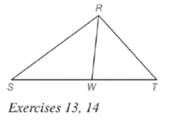Chapter 5.6, Problem 14E### Elementary Geometry for College St...

6th Edition
Daniel C. Alexander + 1 other
ISBN: 9781285195698

#### Solutions

Chapter
Section### Elementary Geometry for College St...

6th Edition
Daniel C. Alexander + 1 other
ISBN: 9781285195698
Textbook Problem
1 views

# Given: R W → bisects ∠ S R T Do the following equalities hold? a) R S S W = R T W T b) m ∠ S = m ∠ TTo determine

a)

To check: Whether the equality RSSW=RTWT holds or not.

Explanation

Given:

RW bisects SRT.

Theorem used:

Angle bisector theorem:

If a ray bisects one angle of a triangle, then it divides the opposite side into segments whose lengths are proportional to the lengths of the two sides that form the bisected angle.

Calculation:

Given: RW bisects SRT

To determine

b)

To check: Whether the equality mS=mT holds or not.

### Still sussing out bartleby?

Check out a sample textbook solution.

See a sample solution

#### The Solution to Your Study Problems

Bartleby provides explanations to thousands of textbook problems written by our experts, many with advanced degrees!

Get Started

#### Use an inverse matrix to solve

Mathematical Applications for the Management, Life, and Social Sciences

#### Evaluate the indefinite integral. dxax+b(a0)

Single Variable Calculus: Early Transcendentals

#### ex(ex)2 = a) ex3 b) e3x c) 3ex d) e4x

Study Guide for Stewart's Single Variable Calculus: Early Transcendentals, 8th